• Slides: 25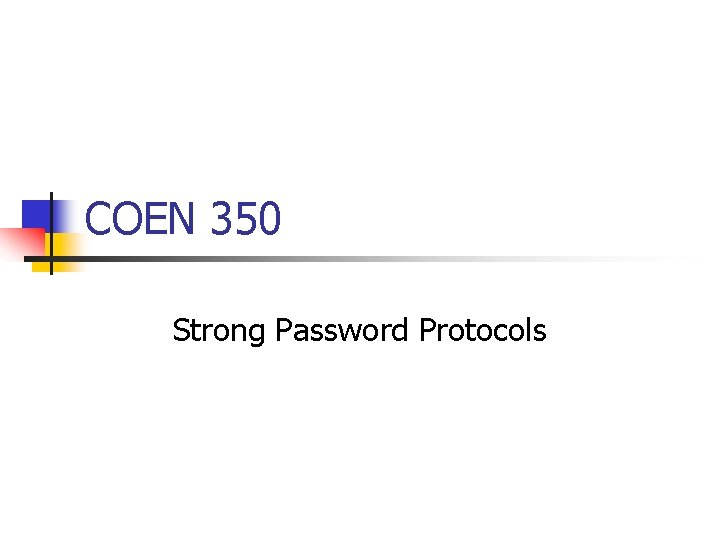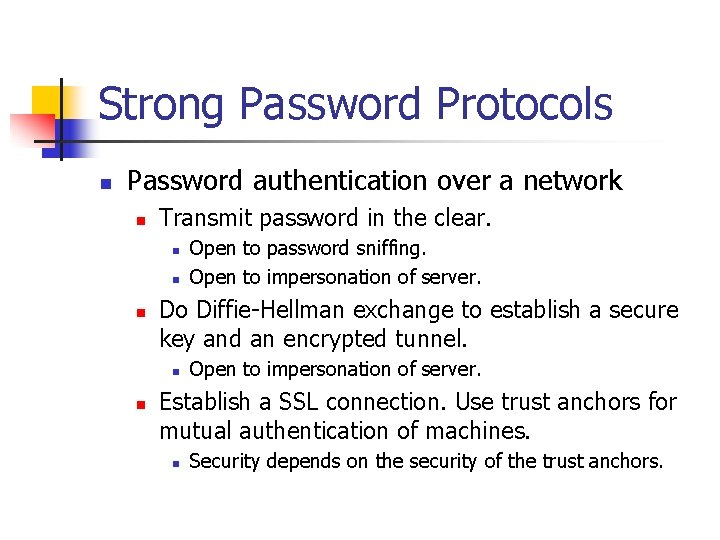Strong Password Protocols n Password authentication over a network n Transmit password in the clear. n n n Do Diffie-Hellman exchange to establish a secure key and an encrypted tunnel. n n Open to password sniffing. Open to impersonation of server. Establish a SSL connection. Use trust anchors for mutual authentication of machines. n Security depends on the security of the trust anchors.Strong Password Protocols n Password authentication over a network n Compute a hash of the password. Use the hash as a secret key in a challenge response scheme. n Scheme: n n Alice to Bob: Login request Bob to Alice: Here is challenge R. Alice to Bob: f (hash(password), R) Open to dictionary attack by eavesdropper or someone impersonating Bob.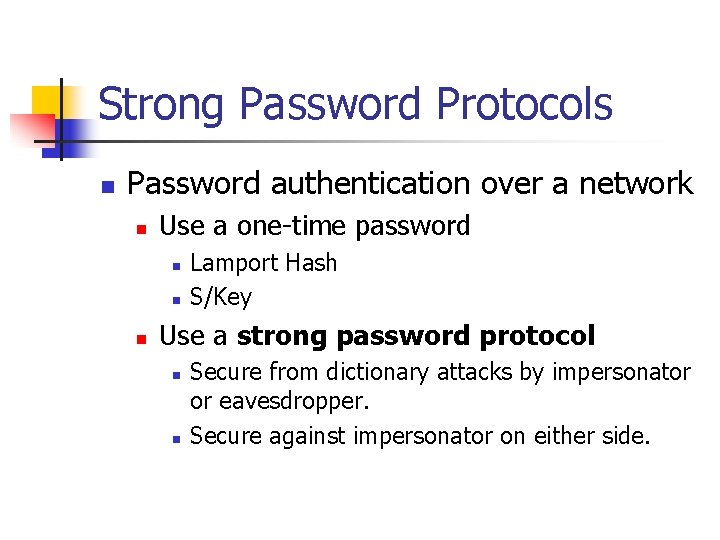Strong Password Protocols n Password authentication over a network n Use a one-time password n n n Lamport Hash S/Key Use a strong password protocol n n Secure from dictionary attacks by impersonator or eavesdropper. Secure against impersonator on either side.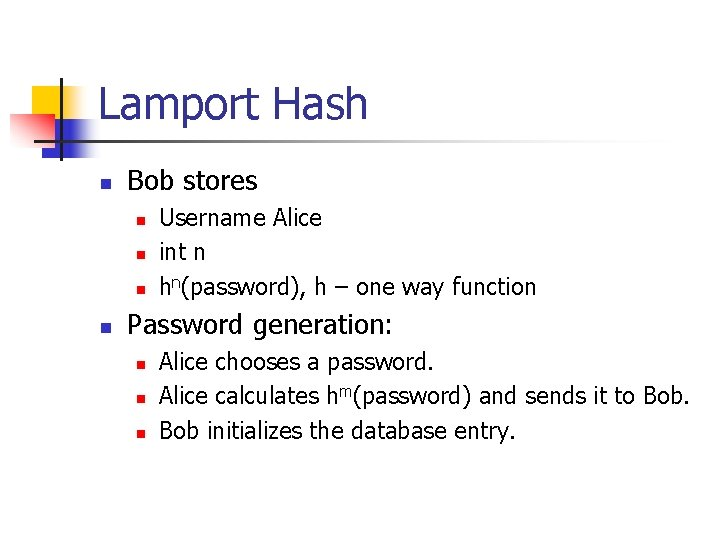Lamport Hash n Bob stores n n Username Alice int n hn(password), h – one way function Password generation: n n n Alice chooses a password. Alice calculates hm(password) and sends it to Bob initializes the database entry.Lamport Hash n Alice Protocol: Alice’s Workstation Alice n hn-1(pwd) Bob password Bob checks: Is h(Alice’s answer) = hn(password). If yes, authenticate. Then replace n with n-1 and store hn-1(password)Lamport’s Hash with Salting n n To prevent password guessing, randomly generate a salt and store it at the server. Calculate hi(pwd, salt)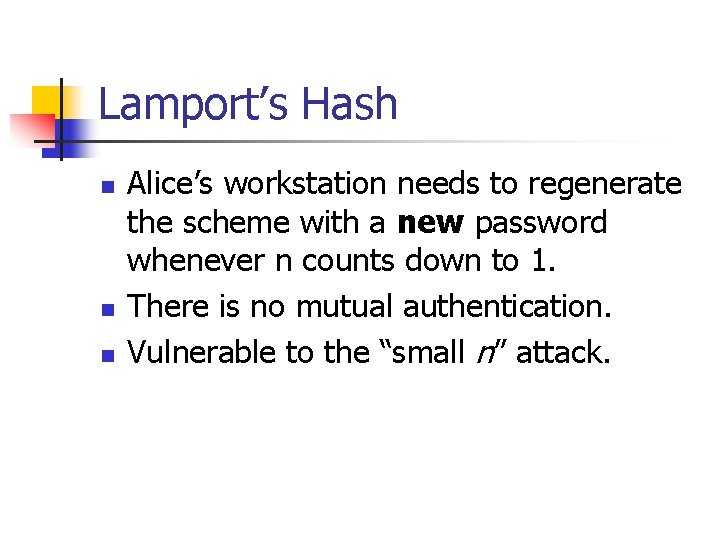Lamport’s Hash n n n Alice’s workstation needs to regenerate the scheme with a new password whenever n counts down to 1. There is no mutual authentication. Vulnerable to the “small n” attack.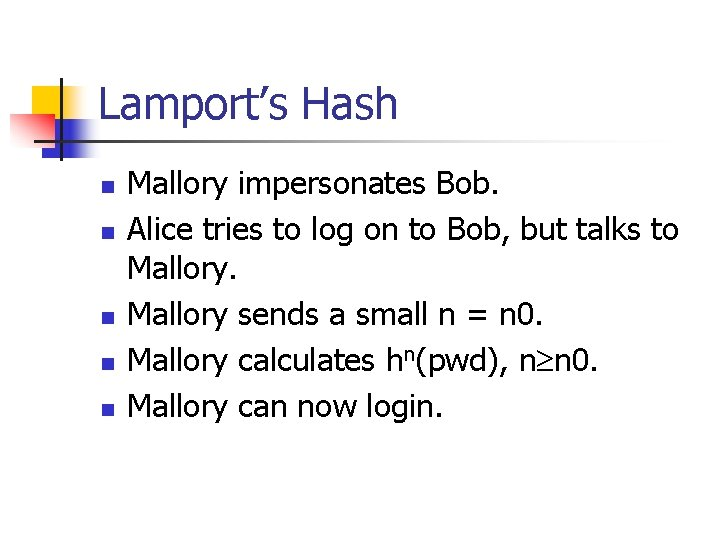Lamport’s Hash n n n Mallory impersonates Bob. Alice tries to log on to Bob, but talks to Mallory sends a small n = n 0. Mallory calculates hn(pwd), n n 0. Mallory can now login.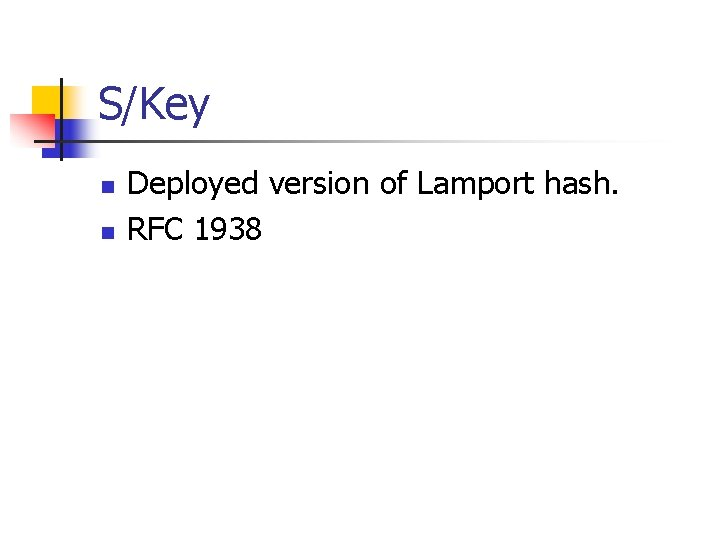S/Key n n Deployed version of Lamport hash. RFC 1938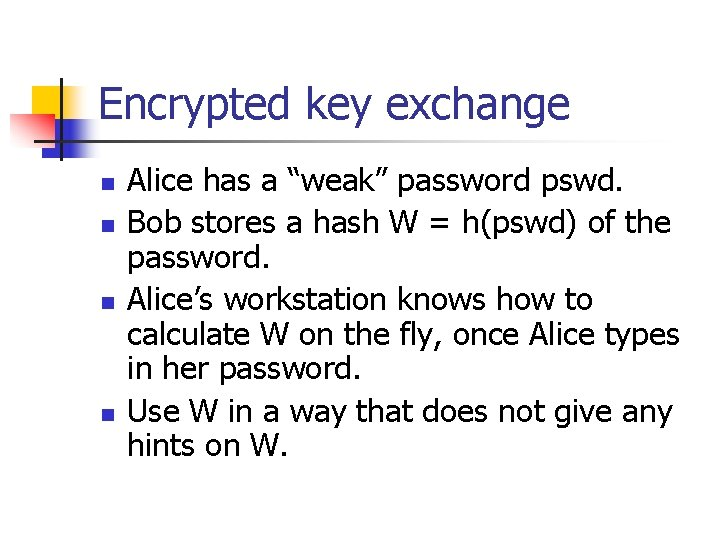Encrypted key exchange n n Alice has a “weak” password pswd. Bob stores a hash W = h(pswd) of the password. Alice’s workstation knows how to calculate W on the fly, once Alice types in her password. Use W in a way that does not give any hints on W.Encrypted key exchange Alice Bob Alice and Bob share a weak secret W = h(password) Alice chooses a random Bob chooses a random b and number a. challenge C 1. Shea sends: Bob encrypts this message and finds. He that Alice has solved his challenge C 1. sends Both Bob and Alice use their knowledge ofa}W to encrypt their Alice, W { g Finally, authenticates himself proves his knowledge of W by Alice then. Bob proves her knowledge of to W Alice. by her. He ability ab. to calculate K. She b mutual messages. They both calculate K = g , C}1}able his picks knowledge of K, which proves to correctly encrypt Alice’s also a challenge C 2 andhesends KW {by C{1 gbeing , C to Bob. 2 challenge C 2. He sends K {C 2 } to Alice.Encrypted key exchange EKE: Diffie-Hellman exchange with encryption. W hash of password. (Bob stores it, Alice can recalculate it). p Modulus. All calculation are based on it. Alice: "Alice", EW(ga) Bob: EW(gb), Challenge CBob At this point, both Alice and Bob calculate K = gab EK(CAlice, CBob) EK(CAlice)EKE: Encrypted Key Exchange n n Secure against eavesdropper because all data are undistinguishable from random numbers. Eavesdropper cannot decide whether the ga, gb are the correct decryption. Secure against impersonation: n If treacherous Trudy impersonates Bob, she guesses a single value W in the first exchange. See the definition again.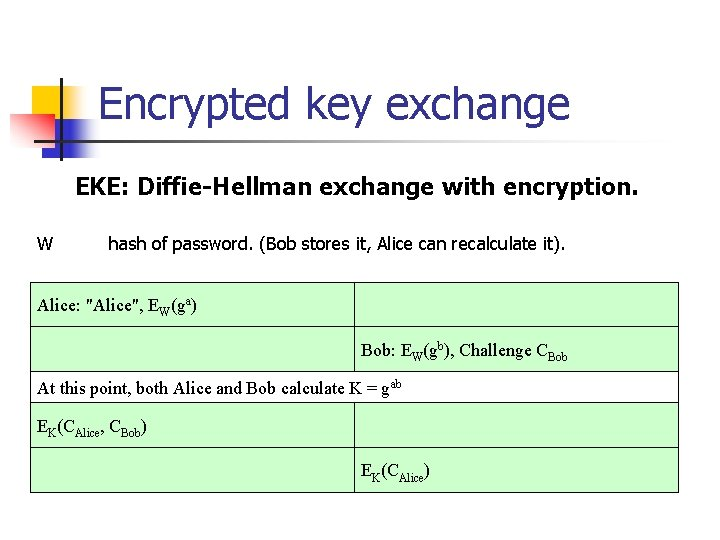Encrypted key exchange EKE: Diffie-Hellman exchange with encryption. W hash of password. (Bob stores it, Alice can recalculate it). Alice: "Alice", EW(ga) Bob: EW(gb), Challenge CBob At this point, both Alice and Bob calculate K = gab EK(CAlice, CBob) EK(CAlice)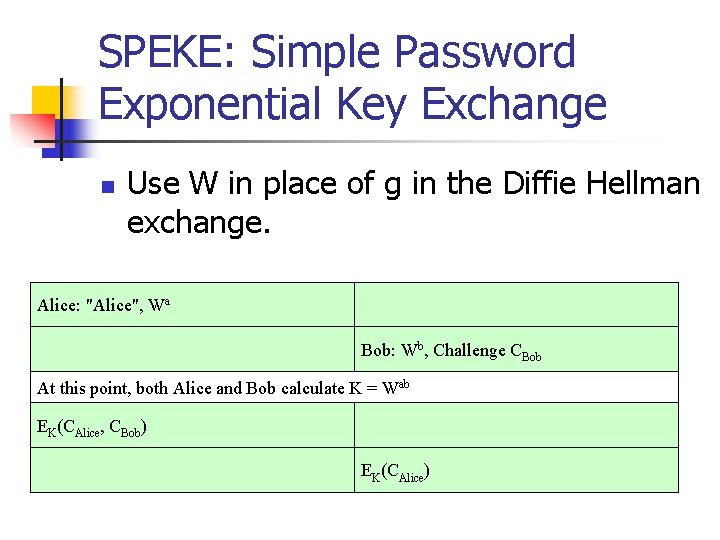SPEKE: Simple Password Exponential Key Exchange n Use W in place of g in the Diffie Hellman exchange. Alice: "Alice", Wa Bob: Wb, Challenge CBob At this point, both Alice and Bob calculate K = Wab EK(CAlice, CBob) EK(CAlice)PDM: Password Derived Moduli n PDM uses modulus p that is a function of the password and uses 2 for g in Diffie Hellman. Alice: "Alice", EW(2 a mod p) Bob: EW(2 b mod p), Challenge CBob At this point, both Alice and Bob calculate K = 2 ab EK(CAlice, CBob) EK(CAlice)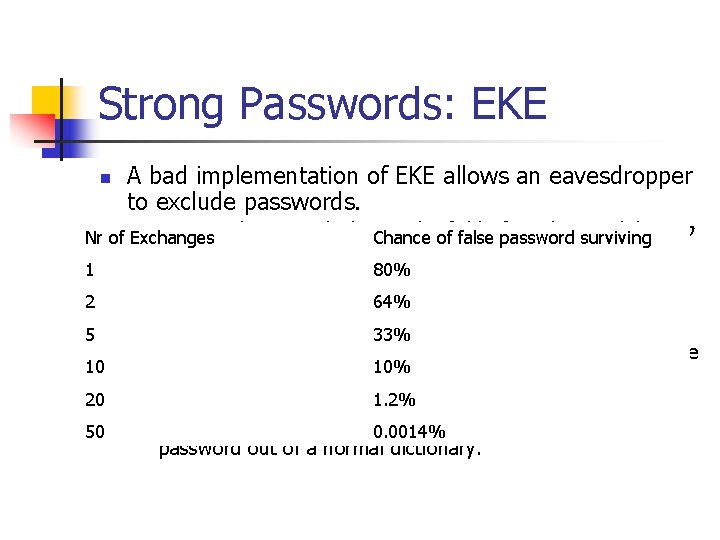Strong Passwords: EKE n A bad implementation of EKE allows an eavesdropper to exclude passwords. n Assume that we calculate in the field of number modulo p, p Nr of Exchanges Chance of false password surviving 1 2 n n 5 10 n 20 n 50 n a prime. Then ga and gb are both 80% m bit numbers smaller than p. 64% Attacker maintains a dictionary of possible passwords and observes many authentication 33% rounds. If W is in the dictionary, he encrypts Alice’s round 1 message M. If W -1{M } > p, then 10% attacker excludes W. 1. 2% Chance of excluding a false password W is 2 m – p / p. If this chance is about 80%, then 50 rounds determine the 0. 0014% password out of a normal dictionary.Augmented Strong Password Protocols n n If someone knows W in EKE, they can impersonate Alice. Augmented Protocols n n n Trudy can steal Bob’s database Trudy can do a dictionary attack. If the dictionary attack is unsuccessful, then she cannot impersonate Alice. n Knowledge of W is not enough.Augmented EKE n Bob stores “Alice, p, g. W mod p” Alice: "Alice", ga mod p Bob: gb + g. W mod p, u, Challenge CBob At this point, both Alice and Bob calculate K = gb(a+u. W) mod p Alice: EK(CBob), CAlice Bob: EK(CAlice)Strong Passwords n Augmented PDM n Server Information Creation n Alice has password pssw Alice sends to Bob n p = f (pssw) [this is a prime] n W = hash (pssw) [one-way hash] Bob stores: n Alice, p, W,Strong Passwords: Augmented PDM Bob Alice chooses creates a random number ba. . a mod p Alice 2 W Bob She calculates recomputes 2 b mod and p. from her password. Alice knows that Bob is Bob because Bob proves that b, hash (2 ab mod p , 2 b. W mod p) to Bob sends 2 b. W he knows 2. Alice 1 now sends Alice hash 2 (2 ab mod p , 2 b. W mod p) Bob knows that Alice is Alice because she proves to him that she knows W. If Alice had just broken into the server, she would have to calculate 2 b. W from 2 W mod p.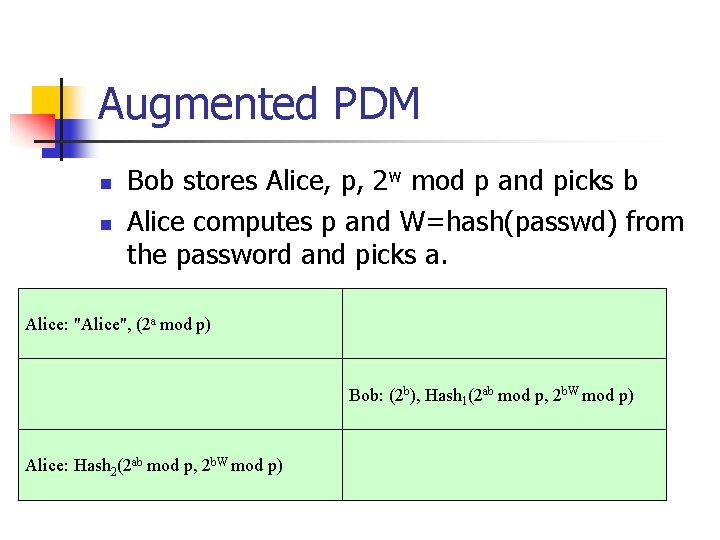Augmented PDM n n Bob stores Alice, p, 2 w mod p and picks b Alice computes p and W=hash(passwd) from the password and picks a. Alice: "Alice", (2 a mod p) Bob: (2 b), Hash 1(2 ab mod p, 2 b. W mod p) Alice: Hash 2(2 ab mod p, 2 b. W mod p)Strong Passwords n Secure Remote Password n n RFC 2945 Bob stores {Alice, g. W mod p}, where W = f (passwd).Strong Passwords SRP Alice creates random a and sends ga to Bob creates random b, challenge CBOB and 32 b number u. Bob sends gb + g. W mod p, u, CBOB to Alice. Both calculate K = g b(a+u. W) mod p. Alice sends K {CBob}, CAlice to Bob sends K {CAlice} to Bob.